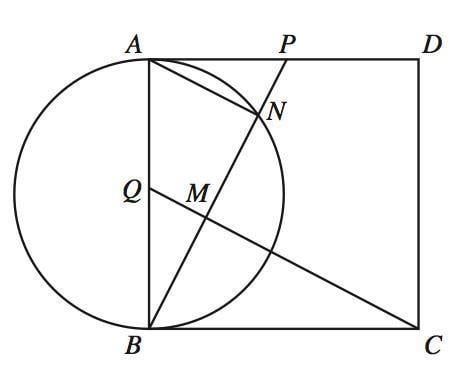Side of a triangle (geometry)

Homework Statement

As shown in the diagram below, the shape consists of a square and a circle with centre Q. Given that QM = 3 cm, prove that MN = 6 cm.

Known data:
-- triangles APB and BQC are congruent
-- angle BMC = 90
-- triangles BMQ and BNA are similar and right-angled2. Homework Equations
3. The Attempt at a Solution

This is a part of a structured question and I've already proved all the data listed above. I also know that AN = 6 cm as the triangles are similar, and I think that the solution might have something to do with triangle AMN being isosceles (but I can't prove that). There's probably something obvious I'm missing, so a nudge in the right direction would be greatly appreciated.

RUber
Homework Helper
Can you show that there is a point X such that ANMX is a square?

Can you show that there is a point X such that ANMX is a square?

Thank you! I think something like this would suffice: Let AX be the line parallel to MN, such that X lies on the extension of CQ. As we know ∠NMQ = 90°, ∠AXM =90° as they are interior angles between parallel lines. Since ∠MNA and ∠XAN are 90° as well, ANMX is a square, and AN = NM. Therefore MN is 6 cm.

Thanks once again.

RUber
Homework Helper
I think that your argument only proves that ANMX is a rectangle. I may have steered you wrong...I do not see a good way to make the conclusion along those lines.
triangles APB and BQC are congruent, so AP = AQ.
So you should be able to show that QMB = NPA.
Then use the isosceles rule to say BN = NM.
And I think you should be able to conclude the result you are looking for.

SammyS
Staff Emeritus
Homework Helper
Gold Member

Homework Statement

As shown in the diagram below, the shape consists of a square and a circle with centre Q. Given that QM = 3 cm, prove that MN = 6 cm.

Known data:
-- triangles APB and BQC are congruent
-- angle BMC = 90
-- triangles BMQ and BNA are similar and right-angled

View attachment 87831

2. Homework Equations
3. The Attempt at a Solution

This is a part of a structured question and I've already proved all the data listed above. I also know that AN = 6 cm as the triangles are similar, and I think that the solution might have something to do with triangle AMN being isosceles (but I can't prove that). There's probably something obvious I'm missing, so a nudge in the right direction would be greatly appreciated.
You should be able to do this using similar triangles.

From the result that AN = 6cm, you can show that ΔAMN is isosceles.

triangles APB and BQC are congruent, so AP = AQ.
So you should be able to show that QMB = NPA.
Then use the isosceles rule to say BN = NM.

You should be able to do this using similar triangles.

From the result that AN = 6cm, you can show that ΔAMN is isosceles.

•